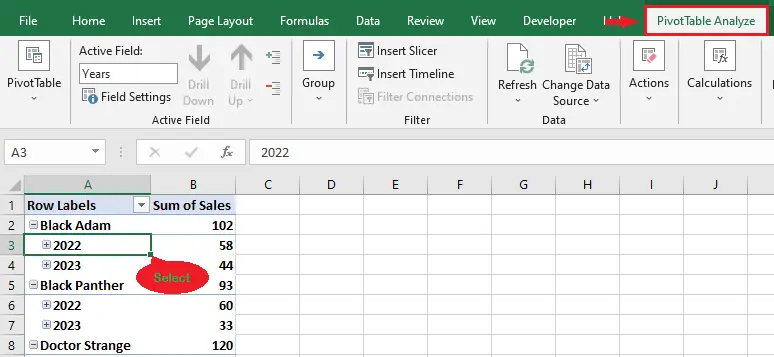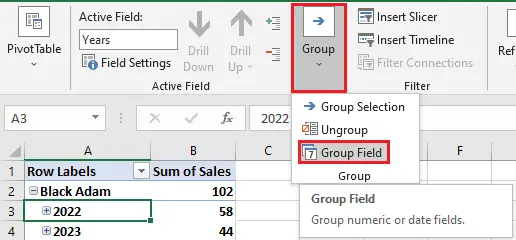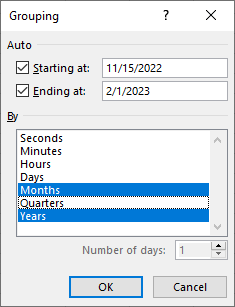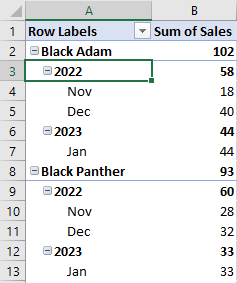# Grouping Excel Pivot Table: Group And Ungroup

Upgrade your Excel skills to the next level by learning how to group a pivot table in Excel. In this article, we will teach you how to group data in a pivot table. Additionally, we will also teach you how to ungroup it if you need to.

Grouping Excel pivot tables can help us quickly summarize and analyze our data. The question is, how do we do that? Read until the end of this article to answer your question.

Let’s first take a quick look at a pivot table before moving on to our tutorial.

## What is a Pivot Table?

pivot table is an essential Excel feature that enables users to organize and summarize complex data easily.

This feature allows users to group their data by columns, sort it by values, and calculate statistics. In addition, pivot tables are handy when you have a large quantity of data.

Now that we already understand the pivot table, let’s finally move on to the tutorial.

### How to group data in Excel Pivot Table

In this example, we are going to group order dates by year and month. See the simple guide below.

1. Click data, then the PivotTable Analyze tab.

First, click on any of the data you want to group, then click the pivot table analyze tab.2. Click the group button, then the group field.

Once the “PivotTable Analyze” tab is selected, click the group button, then select the group field.3. Edit the grouping dialog box as you wish.

After clicking the group field, a grouping dialog box will appear. Edit the grouping dialog box as you wish or as you want your data to be grouped, then click OK.4. Result.``Tip: The keyboard shortcut keys for grouping selected pivot tables are ALT + Shift + (→) Right Arrow Key.``

An alternative way of grouping data in an Excel pivot table

The following is an alternative way of grouping data in the Excel pivot table:

1. Right-click the data, then select the group.
2. After clicking the group button, a grouping dialog box will appear. Edit the grouping dialog box as you wish or as you want your data to be grouped, then click OK.

### Ungroup data in an Excel pivot table

The following are the steps on how to ungroup data in an Excel pivot table:

Step 1: Click the data that’s been grouped.
Step 2: Click the PivotTable Analyze tab, then the group button.
Step 3: Select Ungroup.

``````Tip: The shortcut keyboard keys for ungrouping the grouped pivot table are Alt + Shift + (←) Left Arrow Key.

Also, you can ungroup data in an Excel pivot table by right-clicking the data and selecting ungroup.``````

## Conclusion

In conclusion, grouping a pivot table in Excel makes us quickly summarize and analyze our data. Learning how to do it is an easy thing to do. Just follow the simple steps in the tutorial above.